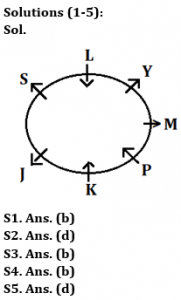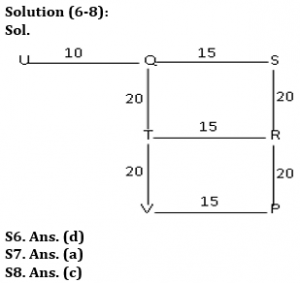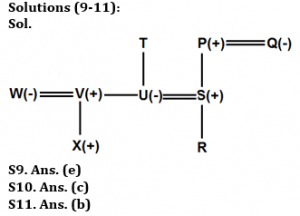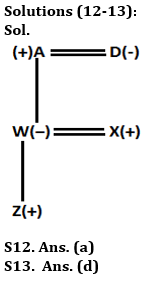Latest Banking jobs   »

# Reasoning Quiz For Bank of Baroda SO 2023 -8th May

Directions (1-5): Study the information carefully and answer the questions given below.
Seven persons are sitting around a circular table. Some of them facing towards the center and some of them facing outside the center. J and K sit immediate left to each other. M sits second to the right of K. Two persons sits between S and M. P sits third to the left of L who is not immediate neighbor of K. Immediate neighbor of L face opposite direction to L. Y face opposite direction to P. Immediate neighbor of Y face opposite direction to each other. Not more than three persons face towards the center.

Q1. Who among the following person sit second to the right of Y?
(a) J
(b) P
(c) S
(d) K
(e) None of these

Q2. How many persons face opposite to the center?
(a) Three
(b) One
(c) Five
(d) Four
(e) None

Q3. How many persons are sitting between S and P, when counted left of P?
(a) Four
(b) Two
(c) Three
(d) More than four
(e) None

Q4. Four of the following five are alike in certain way based from a group, find the one that does not belong to that group?
(a) Y-K
(b) K-P
(c) M-P
(d) L-S
(e) J-L

Q5. What is the position of Y with respect to K?
(a) Fourth to the right
(b) Immediate left
(c) Second to the left
(d) Third to the right
(e) None of these

Directions (6-8): Study the following information carefully and answer the given questions.
Point S is 40 m towards the North of Point P. Point Q is 15 m towards the West of point S. Point U is 25 m towards the West of point S. Point T is 20 m towards the South of point Q. Point R is 15 m towards the East of point T. Point V is 15 m towards the West of point P.

Q6. Which of the following points are in a straight line?
(a) S, T, P
(b) T, V, R
(c) S, Q, V
(d) T, V, Q
(e) U, Q, R

Q7. P is in which direction with respect to R?
(a) South
(b) North
(c) East
(d) West
(e) Cannot be determined

Q8. If a person walks 10 m towards East from point U and then takes a right turn, which of the following points would he reach first?
(a) V
(b) S
(c) T
(d) P
(e) R

Directions (9-11): Study the information carefully and answer the questions given below.
There are nine members in a family in which only three married couples. There are three generation in this family. Q is the wife of P. P is the grandfather of R. Q has only one son who is married to T’s child. T has only two children one son and one daughter. X is grandson of T. S is brother-in-law of son of T. U and V are children of T. W is married to the son of T. X is nephew of U and he is W’s child. U is a married woman.

Q9. If R is married to Y, then how is Y related to S?
(a) Son
(b) Daughter
(c) Son-in-law
(d) Daughter-in-law
(e) Can’t be determined

Q10. How is S related to T?
(a) Son
(b) Daughter
(c) Son-in-law
(d) Daughter-in-law
(e) Husband

Q11. How is Q related to R?
(a) Grandfather
(b) Grandmother
(c) Uncle
(d) Aunt
(e) Either (c) or (d)

Directions (12-13): Study the following information carefully and answer the given questions:
In a family of five members, there are two married couples and three generations in the family. A is father-in-law of X who is married with W. Z is the son of W. D who is a married woman has only one daughter.

Q12. How is A related with Z?
(a) Grand Father
(b) Grand Mother
(c) Father-in-law
(d) Daughter in law
(e) Grandson

Q13. Who is the son-in-law of Z’s grandmother?
(a) A
(b) D
(c) W
(d) X
(e) None of these

Q14. How many pairs of letters are there in the word “ADEQUATE” each of which have as many letters between them in the word as they have between them in the English alphabetical series (both forward and backward direction)?
(a) Three
(b) One
(c) Two
(d) More than three
(e) None

Q15. If in the number 63143214, add 1 after multiplying by 2 in each of the odd digit and subtract 3 after multiplying by 2 in each of the even digit then, how many digits are repeating in new number?
(a) Three
(b) One
(c) None
(d) Two
(e) Four

SolutionsS14. Ans. (c)
Sol. DE, QT

S15. Ans. (a)
Sol. Original number- 63143214
Obtained number- 97357135
Repeating numbers= 3, 5 and 7## FAQs

### What is the selection process of Bank of Baroda SO 2023?

The selection process of Bank of Baroda SO consists of Online exam and Interview

#### Congratulations!Union Budget 2023-24: Free PDF